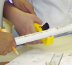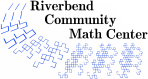# Plumbing and Pipe-Fitting Challenges

### Description

Students often wonder when they will use the math they learn in school. These activities are designed to answer that question as it relates to measuring, working with fractions and decimals, basic geometry, and the Pythagorean Theorem. Plumbers and pipe-fitters need to have a good working knowledge of these and other math concepts to solve problems that arise frequently on the job. To enter any of the building trades, an apprentice must score well on basic math tests. A solid understanding of basic math opens the door to many financially and personally rewarding careers.

1 to 2 hours

### Activity Time

60 to 90 minutes for each day -- the activity is designed to last at least two days. Activity can be extended into sequence of classes.

### Topics

• Measuring lengths to the nearest 1/8 and 1/16
• Converting fractions to decimals
• Adding and subtracting fractions and mixed numbers
• Using benchmark fractions
• Simplifying fractions
• Approximating irrational numbers to the nearest eighth
• Pythagorean Theorem
• Recognizing angles

### Goals

• Students will experience problem solving situations that motivate them to measure and compute accurately
• Students will improve their fluency with problem solving involving fractions and decimals
• Students will practice using the Pythagorean Theorem

### Materials

• FOR 30 STUDENTS
• 30 Handouts
• Blank paper (30 sheets in case students want to design their own blueprints)
• Saran wrap for wrapping pipes
• 6 team bags for fittings and small pipes
• 30 Pencils
• 30 Rulers
• 15 Tape measures
• 15 Pipe cutters
• 28 5-foot length PVC pipes (3/4" diameter)
• 300 90 degree elbow connectors (3/4")
• 300 45 degree connectors (3/4")
• 300 T connectors (3/4")
• 30 straight connectors (3/4")
• Digital camera (so students can record their achievements)
• First Aid Kit
• Posterboard templates for checking models
• 12 to 24 pipe holders with clamps (Day 2)
• 6 Screwdrivers (Day 2)
• 6 Screw-in clamps (Day 2)
• 6 Wooden frames (Day 2)
• 1 Roll of masking tape (Day 2)
• 6 Styrofoam or cardboard boxes (Day 2)
• 6 Calculators (Day 2)

### Prerequisites

• Exposure to fraction equivalence
• Exposure to addition and subtraction of mixed numbers
• Exposure to converting fractions to decimals
• Exposure to measuring lengths to the nearest 1/8 of an inch
• Familiarity with angles

• Computing with the Pythagorean Theorem

### Credits

Many thanks to Robin Forsythe and other members of Plumbers and Pipefitters Local Union 172 for their help in developing and field testing this activity

### Author

Amanda Katharine Serenevy

### National Common Core Standards

 5.Focus.1 Develop fluency with addition and subtraction of fractions, and develop understanding of multiplication of fractions and division of fractions in limited cases. 5.NBT.4 Use place value understanding to round decimals to any place. 5.NF.1 Add and subtract fractions with unlike denominators (including mixed numbers) by replacing given fractions with equivalent fractions in such a way as to produce an equivalent sum or difference of fractions with like denominators. 5.NF.3 Interpret a fraction as division of the numerator by the denominator. Solve word problems involving division of whole numbers leading to answers in the form of fractions or mixed numbers, e.g. by using visual fraction models or equations to represent the problem. 5.NF.7 Apply and extend previous understandings of division to divide unit fractions by whole numbers and whole numbers by unit fractions. 6.NS.1 Interpret and compute quotients of fractions and solve word problems involving division of fractions by fractions. 7.NS.1 Apply and extend previous understandings of addition and subtraction to rational numbers, and represent addition and subtraction on a number line. 7.NS.3 Solve real-world problems involving the four operations with rational numbers. 8.G.7 Apply the Pythagorean Theorem to determine unknown side lengths in right triangles in real-world and mathematical problems in two and three dimensions. 8.NS.1 Know the difference between rational and irrational numbers, and show that the decimal expansion for rational numbers repeats or terminates. Convert repeating decimals into rational numbers. 8.NS.2 Use rational approximations of irrational numbers to compare the size of irrational numbers, locate them approximately on a number line, and estimate the value of expressions involving irrationals.
Riverbend Community Math Center
hello@riverbendmath.org
http://riverbendmath.org
(574) 339-9111This work placed into the public domain by the Riverbend Community Math Center.